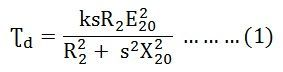# Maximum Torque Condition of an Induction Motor

In the article named Torque Equation of an Induction motor, we have seen the developed torque and its equation. Here, the Maximum Torque Condition of an induction motor is discussed. The torque produced in the induction motor mainly depends on the following three factors. They are the strength of the rotor current; the flux interacts between the rotor of the motor and the power factor of the rotor. The value of torque when the motor is running is given by the equation shown below:The total impedance of the RC network always lies between and 90º. The impedance is the opposition offered by the electronic circuit element to the flow of current. If the impedance of the stator winding is assumed to be negligible. Thus, for the given supply voltage V1, E20 remains constant.The developed torque will be maximum when the right-hand side of the equation (4) will be maximum. This condition is possible when the value of the denominator shown below is equal to zero.

Hence, the developed torque is maximum when the rotor resistance per phase is equal to the rotor reactance per phase under running conditions. By putting the value of sX20 = R2 in equation (1) we get the equation for maximum torque.The above equation shows that the maximum torque is independent of the rotor resistance.

If sM is the value of slip corresponding to the torque which is maximum then from the equation (5)Therefore, The speed of the rotor at maximum torque is given by the equation shown below.The following conclusion about the maximum torque can be drawn from equation (7) are given below.

• It is independent of the rotor circuit resistance.
•  Torque at the maximum condition varies inversely as the standstill reactance of the rotor. Hence, for maximum torque, X20 and therefore, the inductance of the rotor should be kept as small as possible.
• By varying the resistance in the rotor circuit, maximum torque can be obtained at any desired slip or speed. It depends upon the rotor resistance at the slip (sM = R2/X20).

To develop maximum torque at the standstill condition, the rotor resistance must be high and should be equal to X20. But to develop a torque that is maximum at the running condition the rotor resistance must be low.